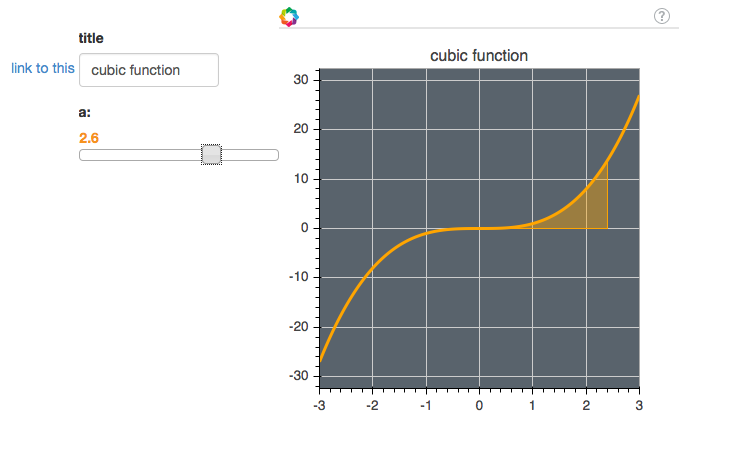# What am I doing wrong in updating my Bokeh figure with patches

I’ve written a Bokeh script which plots a cubic function on (-3,3) and fills in a variable part of it with patches with

``````    px = x[N//2:N//2+a*100]
px = np.append(px, px[::-1])
py = y[N//2:N//2+a*100]
py = np.append(py, np.zeros(len(px//2)))
print(type(self.p1))
self.p1.patch(px, py, color='orange', fill_alpha=0.4)
``````

This works when the figure is first rendered by bokeh-server, but when I slide my variable (a), nothing is updated and I get the error:

AttributeError: ‘Plot’ object has no attribute ‘patch’

<class ‘bokeh.plotting.Figure’>
to being
<class ‘bokeh.models.plots.Plot’>

and a Plot object is no longer something I can draw patches on.

What is the correct way to do this, please? (Full code at http://pastebin.com/0ZX4LfHF)

Best wishes,

Christian···

From my print statement in the above snippet it seems as though self.p1 has changed from being